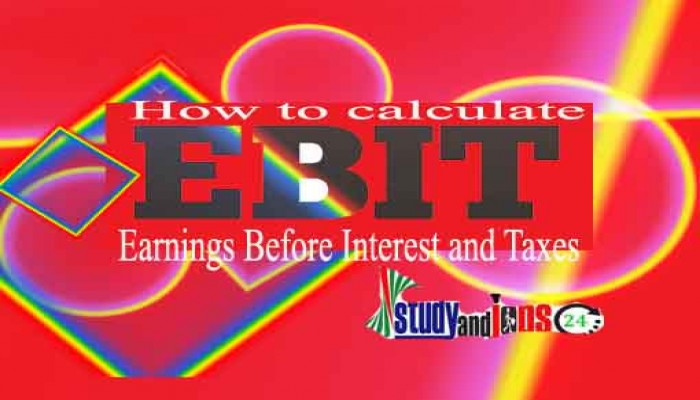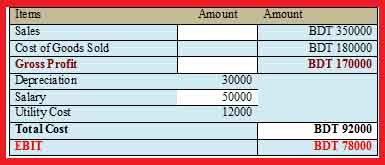Zulfiqar Hasan

EBIT is an important tool to measure the initial profitability of a business organization. EBIT is an indicator of a company's profitability, calculated as revenue minus expenses, excluding tax and interest. EBIT is also referred to as "operating earnings", "operating profit" and "profit before interest and taxes (PBIT).

In finance, earnings before interest and taxes, is a measure of a company's benefit that precludes interest and income tax expenses. EBIT is the difference between working incomes and working liabilities. It can be calculated in Tabular format or by using and algebraic formula. Following examples will explain it.

Example 01: Calculate EBIT from the following information. Sales BDT 350000, Cost of Goods Sold BDT 180000, Depreciation BDT 30000, Salary BDT 50000, Utility Cost BDT 12000.

Solution: Tabular FormatEBIT = Gross Profit - Total Cost

Algebraic Formula for EBIT

EBIT also can be calculated by using algebraic formula.

EBIT=(PxQ) - FC - (VCxQ)

where,

P  = sales price per unit

Q  = sales quantity

FC = total fixed operating costs

VC = variable operating costs per unit

and Sales = PxQ

Example 02:

Suppose a firm has \$10000 in fixed operating costs, its price per unit for its product is \$10 and its variable costs per unit are \$8. Suppose your initial sales quantity is 8000 units. Find out EBIT?

Solution:

EBIT=(PxQ)-FC-(VCxQ)

=(\$10X8000)-\$10000-(\$8X8000)

=\$6000

Contributor: Zulfiqar Hasan is working as an Associate Professor of Finance. He can be reached: hasanzulfiqar@yahoo.co.uk